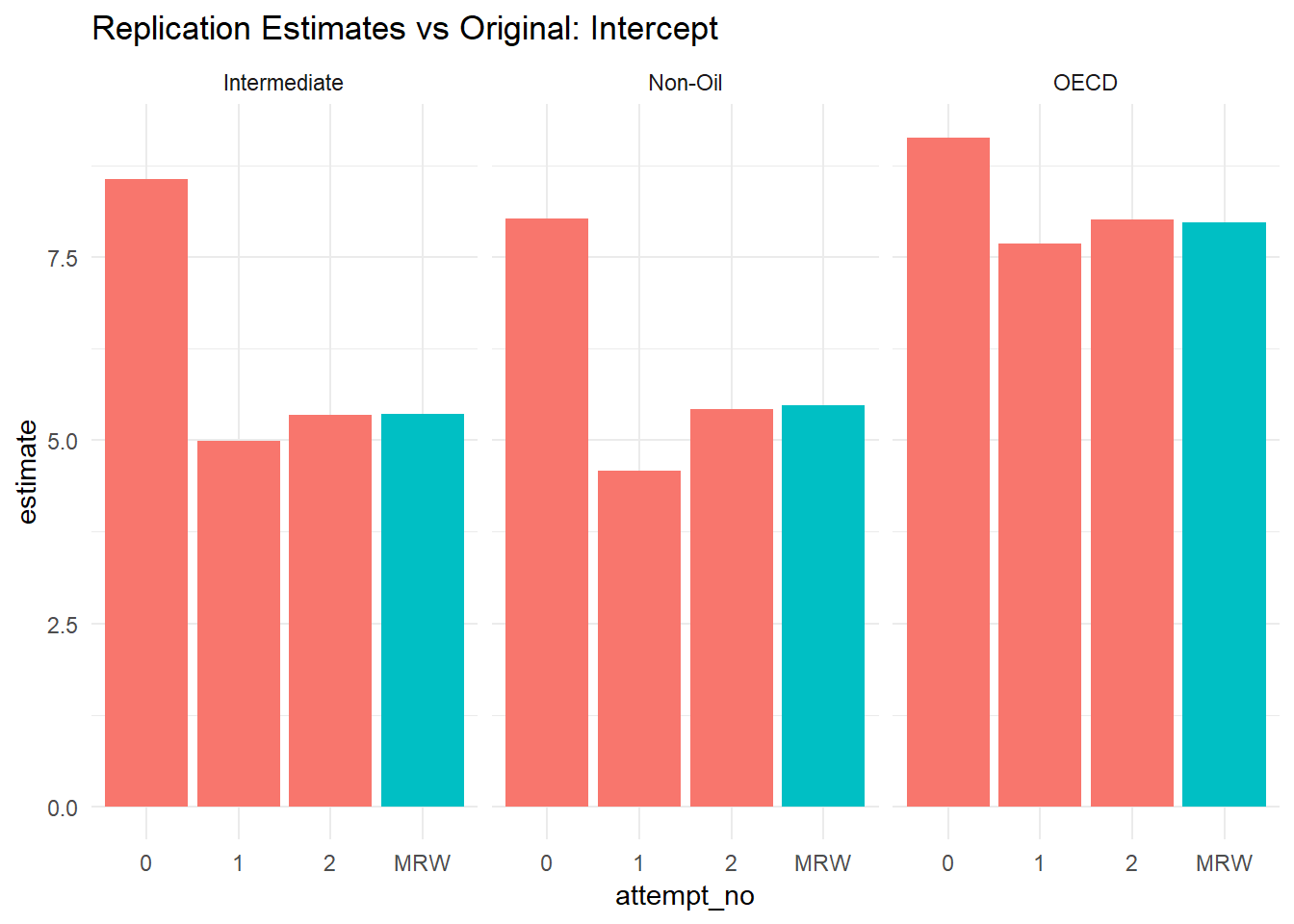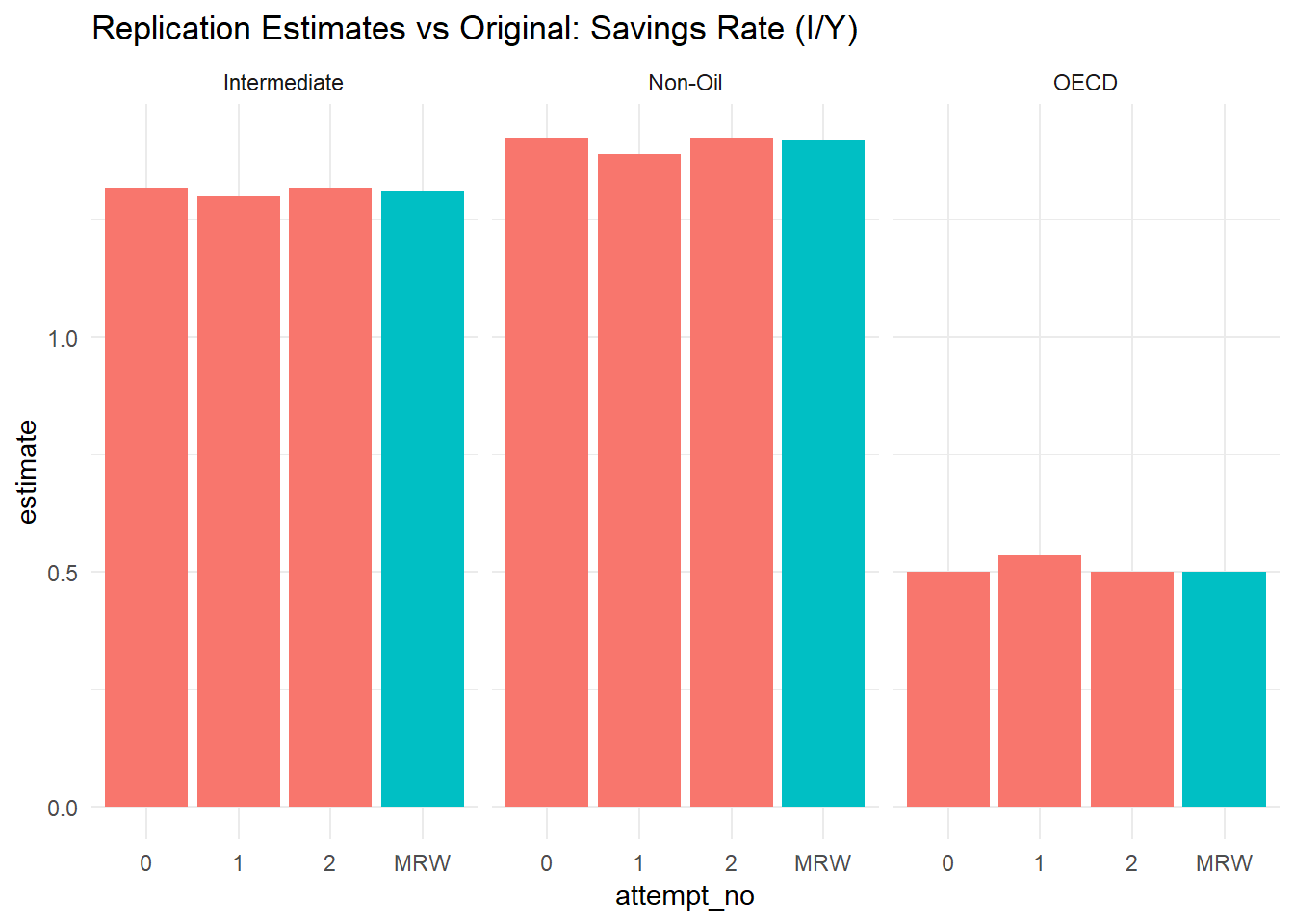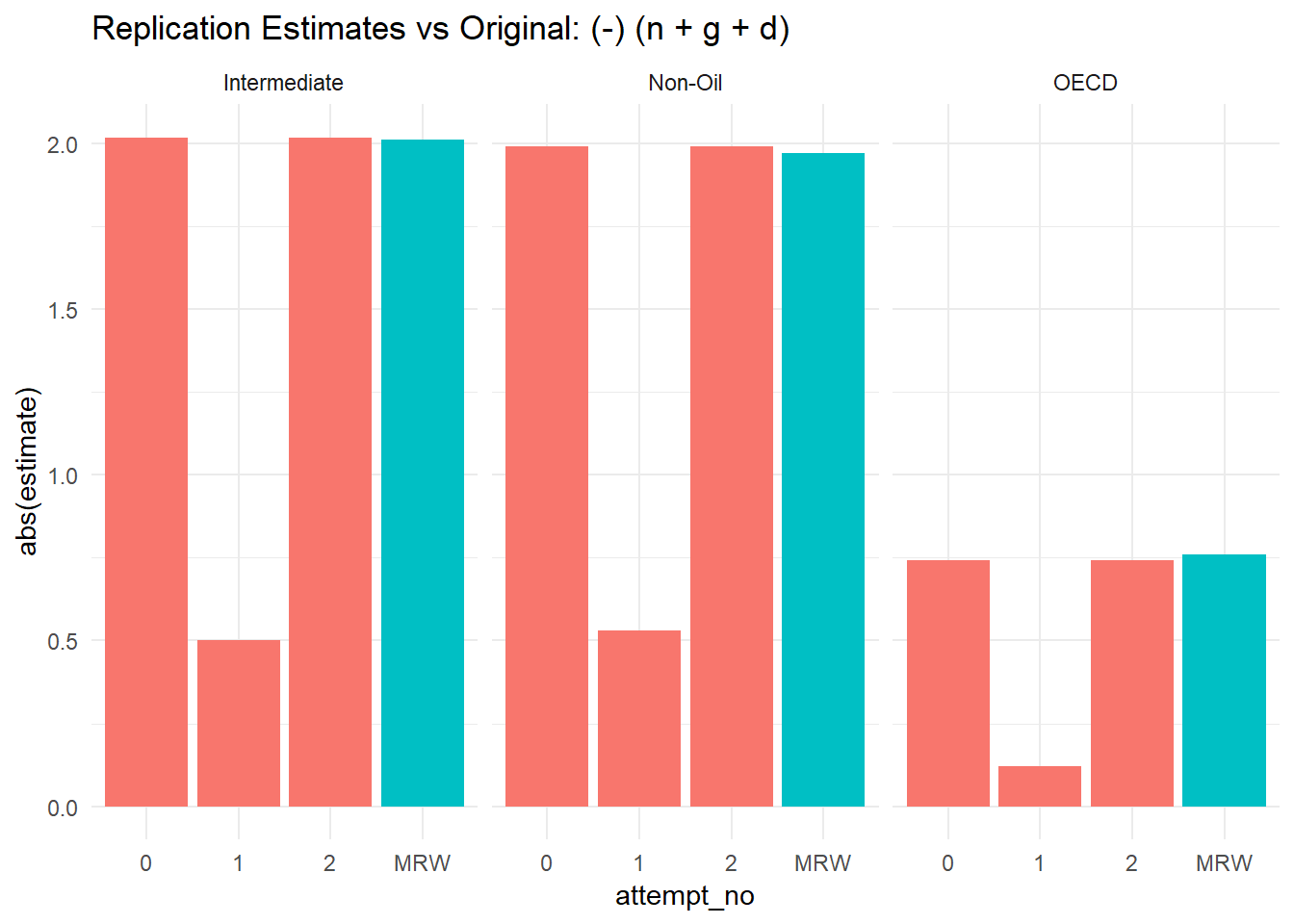In this post I finally solve the replication issues that plagued my last attempt. In hindsight, the naive addition of +5 to the working_age_pop_ch variable makes me feel a little foolish although the simulated histograms at the bottom of this post look quite pretty, so maybe it balances out?

## Re-Parametrisation

As discussed in the conclusion of my last post, the difference in replicated intercepts could be because of incorrect parametrisation on my part. I argued that it wasn’t entirely clear how the variables $n + g + d$ (and by extension $s$) should be parametrised and indeed whether the appendix data is already in the required format - this is exacerbated by the lack of units in the appendix - to combat this I have two alternative approaches that make sense to me:

The first uses $g_i + d_i = 0.05$ without any percentage transformation i.e. identical to the 0.05 value discussed by MRW in the paper’s body and the second converts $s$ and $n$ from (presumably percentage values) to fractions.

Below I transform the data and use purrr’s map function to run the regression on both re-parametrised datasets in turn, then I collect all the results in one tidy(ish) tibble using the collect.results function defined previously:

library(readr)
library(dplyr)
library(purrr)
library(broom)
col_types = cols(col_factor(NULL), col_double(), col_double(), col_factor(NULL)))

trial_0 <- MRW_clean

trial_1 <- MRW_clean %>%
mutate(n_g_d = working_age_pop_ch + 0.05)

trial_2 <- MRW_clean %>%
mutate(n_g_d = (working_age_pop_ch + 5) / 100,
s = s / 100)

solow_formula <- log(1985) ~ log(s) + log(n_g_d)

## Non-Oil

re_parametrisation_fits_non_oil <- list(trial_0,
trial_1,
trial_2) %>%
map(~lm(data = filter(., N == 1 & !is.na(school)),
formula = solow_formula))

## Intermediate

re_parametrisation_fits_intermediate <- list(trial_0,
trial_1,
trial_2) %>%
map(~lm(data = filter(., I == 1 & !is.na(school)),
formula = solow_formula))
## OECD

re_parametrisation_fits_oecd <- list(trial_0,
trial_1,
trial_2) %>%
map(~lm(data = filter(., O == 1 & !is.na(school)),
formula = solow_formula))

re_param_fits_all <-  c(re_parametrisation_fits_non_oil,
re_parametrisation_fits_intermediate,
re_parametrisation_fits_oecd)

re_parametrisation_results <- re_param_fits_all %>%
collect.results(models = ., names = list('Non-Oil 0',
'Non-Oil 1',
'Non-Oil 2',
'Intermediate 0',
'Intermediate 1',
'Intermediate 2',
'OECD 0',
'OECD 1',
'OECD 2'))

knitr::kable(head(re_parametrisation_results, 10))
termestimatestd.errorstatisticp.valuesubset
(Intercept)8.03530511.27892856.2828410.0000000Non-Oil 0
log(s)1.42401430.14310589.9507790.0000000Non-Oil 0
log(n_g_d)-1.98977450.5633619-3.5319650.0006385Non-Oil 0
(Intercept)4.58897720.432179710.6182160.0000000Non-Oil 1
log(s)1.38811140.14158569.8040420.0000000Non-Oil 1
log(n_g_d)-0.53008610.1297557-4.0852610.0000919Non-Oil 1
(Intercept)5.42988311.58388993.4281950.0009000Non-Oil 2
log(s)1.42401430.14310589.9507790.0000000Non-Oil 2
log(n_g_d)-1.98977450.5633619-3.5319650.0006385Non-Oil 2
(Intercept)8.56785801.30505406.5651370.0000000Intermediate 0

Now comparing the intercepts:

library(tidyr)
library(ggplot2)

comparison_tbl <- bind_rows(re_parametrisation_results %>% select(term, estimate, subset) %>% mutate(type = 'replication'),
solow_MRW %>% select(term, estimate, subset) %>% mutate(subset = paste0(subset, ' MRW'),
type = 'original')) %>%
separate(col = subset,
into = c('subset', 'attempt_no'),
sep = ' ')

comparison_intercept <- comparison_tbl %>%
filter(term == '(Intercept)')

ggplot(comparison_intercept, aes(x = attempt_no, y = estimate,  fill = ifelse((attempt_no == 'MRW'), 'red', 'blue'))) +
guides(fill = 'none') +
geom_col() +
guides(size = 'none', colour = 'none') +
facet_wrap(~subset) +
theme_minimal() +
ggtitle(label = 'Replication Estimates vs Original: Intercept')From the above it seems certainly possible that the interpretation of $g_i + d_i$ in the first post was very off and the second attempt, rewriting each term as a fraction, is correct. Fortunately, this holds for the other variables as well:

comparison_s <- comparison_tbl %>%
filter(term == 'log(s)')

ggplot(comparison_s, aes(x = attempt_no, y = estimate,  fill = ifelse((attempt_no == 'MRW'), 'red', 'blue'))) +
guides(fill = 'none') +
geom_col() +
guides(size = 'none', colour = 'none') +
facet_wrap(~subset) +
theme_minimal() +
ggtitle(label = 'Replication Estimates vs Original: Savings Rate (I/Y)')comparison_n_g_d <- comparison_tbl %>%
filter(term == 'log(n_g_d)')

ggplot(comparison_n_g_d, aes(x = attempt_no, y = abs(estimate),  fill = ifelse((attempt_no == 'MRW'), 'red', 'blue'))) +
guides(fill = 'none') +
geom_col() +
guides(size = 'none', colour = 'none') +
facet_wrap(~subset) +
theme_minimal() +
ggtitle(label = 'Replication Estimates vs Original: (-) (n + g + d)')Using the comparison function created last time:

re_param_comparison <- list('(Intercept)',
'log(n_g_d)',
'log(s)') %>%
map_df(compare.results,
parameter_type = 'estimate',
results_replicated = re_parametrisation_results %>%
separate(subset, into = c('subset', 'attempt_no'), sep = ' ') %>%
filter(attempt_no == '2') %>%
select(-attempt_no),
results_original = solow_MRW)

knitr::kable(re_param_comparison)
termsubsetestimate_replicatedestimate_originaldiffrounded_diffpct_orig
(Intercept)Non-Oil5.42988315.480.05011690.050.9145420
(Intercept)Intermediate5.34586525.360.01413480.010.2637088
(Intercept)OECD8.02060687.97-0.0506068-0.05-0.6349656
log(n_g_d)Non-Oil-1.9897745-1.970.01977450.02-1.0037809
log(n_g_d)Intermediate-2.0171995-2.010.00719950.01-0.3581838
log(n_g_d)OECD-0.7419215-0.76-0.0180785-0.022.3787554
log(s)Non-Oil1.42401431.42-0.00401430.00-0.2826960
log(s)Intermediate1.31755271.31-0.0075527-0.01-0.5765386
log(s)OECD0.49988950.500.00011050.000.0220925
knitr::kable(re_param_comparison %>%
group_by(term) %>%
summarise(mean(diff)))
termmean(diff)
(Intercept)0.0045483
log(n_g_d)0.0029651
log(s)-0.0038188

There’s pretty conclusive evidence that my first argument was in fact correct and not my second, favoured, hypothesis. However, there’s still room to improve the replication and test whether the small differences between replication and original estimates are driven by rounding errors in our (now) correct model.

## Rounding Errors

If we look at working_age_pop_chh, s and school as well as 1985 it’s clear that the variables have been recorded in the appendix to one decimal place and as an integer respectively. For instance, India’s working_age_pop_ch could plausibly be anywhere from 2.35 to 2.45 in MRW’s original dataset.

MRW_clean %>%
filter(number == 49) %>%
knitr::kable()
numbercountryNIO19601985gdp_chworking_age_pop_chsschooln_g_d
49India11097813393.62.416.85.17.4

To explore whether this imprecision is responsible for the small differences in our estimates we can sample from a distribution taking values between -0.05 and +0.05 (or +/- 0.5 for the GDP figure) and add this random variable to each of our observations that we think have been rounded. I’ll start with the uniform distribution since I have no priors on the distribution of observations at the hundredth level:

create.rvs <- function(data, subset, dummy_argument){
subset <- enquo(subset)
n <- nrow(data)

gdp_rv <- runif(n = n,
max = 0.5,
min = -0.5)
n_rv <- runif(n = n,
max = 0.05,
min = -0.05)
s_rv <- runif(n = n,
max = 0.05,
min = -0.05)

data$1985 <- data$1985 + gdp_rv
data$working_age_pop_ch <- data$working_age_pop_ch + n_rv
data$s <- data$s + s_rv
data <- data %>%
mutate(n_g_d = (working_age_pop_ch + 5) / 100,
s = s / 100) %>%
filter(!!subset == 1 & !is.na(school))

return(data)
}

The create.rvs function is pretty self-explanatory apart from maybe enquo and !! - these let our function arguments play nicely with dplyr’s quasiquotation.1 The last few lines of the function ensure we’re using the correct parametrisation of s and n_g_d as well as filtering and removing missing school observations and finally dummy_argument is a quick work around to make map easier to run N times.

Next, we generate 10,000 simulations of the data and calculate the corresponding regression coefficients. Finally, we collect our results and gather into a long format to make plotting easier (this lets us use facet_grid(subset ~ term) later).

Ideally when generating such a large number of draws we’d use some form of parallel processing as this is an example of an embarrassingly parallel problem.2 Fortunately, Davis Vaughan has recently developed furrr, a parallel implementation of map using the R’s future library. Unfortunately, I ran into an issue with dplyr’s quasiquotation and future_map so the current code uses the traditional map.3

library(furrr)
plan(multiprocess)

rv_non_oil <- 1:10000 %>%
future_map(~create.rvs(data = MRW_clean, subset = N)) %>%
future_map_dfr(~lm(data = .,
formula = solow_formula)$coefficients %>% bind_rows) %>% mutate(subset = 'Non-Oil') rv_intermediate <- 1:10000 %>% future_map(~create.rvs(data = MRW_clean, subset = I)) %>% future_map_dfr(~lm(data = ., formula = solow_formula)$coefficients %>%
bind_rows) %>%
mutate(subset = 'Intermediate')

rv_oecd <- 1:10000 %>%
future_map(~create.rvs(data = MRW_clean, subset = O)) %>%
future_map_dfr(~lm(data = .,
formula = solow_formula)$coefficients %>% bind_rows) %>% mutate(subset = 'OECD') rv_tbl_wide <- bind_rows(rv_non_oil, rv_intermediate, rv_oecd) rv_tbl <- rv_tbl_wide %>% gather(term, estimate, -subset) Originally these plots used facet_grid but even with space = 'free' and scales = 'free' the axes weren’t very appealing so instead we use plotly’s subplot feature: sliderInput('simulation_n', label = 'Simulation Draws', min = 1, max = 10000, value = 8000) library(plotly) renderPlotly({ p_non_oil <- ggplot(rv_tbl %>% filter(subset == 'Non-Oil') %>% group_by(term) %>% top_n(input$simulation_n), aes(estimate, fill = term)) +
geom_histogram(binwidth = 0.005) +
geom_vline(data = solow_MRW %>%
filter(subset == 'Non-Oil'), aes(xintercept = estimate)) +
guides(fill = FALSE) +
theme_minimal() +
theme(legend.position="none") +
facet_wrap(~term,
scales = 'free')

p_intermediate <- ggplot(rv_tbl %>%
filter(subset == 'Intermediate') %>%
group_by(term) %>%
top_n(input$simulation_n), aes(estimate, fill = term)) + geom_histogram(binwidth = 0.005) + geom_vline(data = solow_MRW %>% filter(subset == 'Intermediate'), aes(xintercept = estimate)) + guides(fill = FALSE) + theme_minimal() + theme(legend.position="none") + facet_wrap(~term, scales = 'free') p_oecd <- ggplot(rv_tbl %>% filter(subset == 'OECD') %>% group_by(term) %>% top_n(input$simulation_n), aes(estimate, fill = term)) +
geom_histogram(binwidth = 0.005) +
geom_vline(data = solow_MRW %>% filter(subset == 'OECD'), aes(xintercept = estimate)) +
guides(fill = FALSE) +
theme_minimal() +
theme(legend.position="none") +
facet_wrap(~term,
scales = 'free')

p_non_oil <- ggplotly(p_non_oil) %>%
layout(yaxis = list(title = 'Non-Oil'))
p_intermediate <- ggplotly(p_intermediate) %>%
layout(yaxis = list(title = 'Intermediate'))
p_oecd <- ggplotly(p_oecd) %>%
layout(yaxis = list(title = 'OECD'))

subplot(p_non_oil, p_intermediate, p_oecd, nrows = 3, margin = 0.05, titleY = TRUE, shareX = FALSE, shareY = FALSE) %>%
layout(title = 'Distribution of Replications with Random Noise vs Original Estimates')
})  

renderPlotly and sliderInput are Shiny functions that make the histogram draw numbers interactive.

Initially I was slightly concerned that the results were so off at what we’d normally consider a large number of draws such as three or four thousand. On reflection I think this is only natural. If this were a machine learning problem we’d use grid search to optimise a number of hyper parameters, usually three or four, in this instance the data are the hyper parameters so it’s hardly surprising that so many draws are required.

## Conclusion

From the graphs it seems pretty clear that imprecision can account for the replicated differences although this is by no means conclusive evidence. Looking at these plots reminds me of two things:

• First, the idea of ‘Bayesian’ test statistics.4 Bayesian test statistics involve sampling from the posterior distribution (posterior predictive checking), calculating sample statistics and comparing with known statistics of the original data - i.e. do our samples replicate the mean, median and mode of the observed dependent variable for example. In our case we’re doing something that’s a bit of a mish-mash, we’re effectively adding a noise variable with a uniform likelihood to a frequentist regression model. I’m pretty sure this is frowned upon as a horrendous butchering of both frequentist and Bayesian econometrics which is an impressive feat in and of itself.

• Secondly, the Tobit model.5 We’re effectively observing censoring in a range from +/- 0.05 of an observation to its closest tenth. Modelling that seems like an incredible headache for little perceivable gain although it’d be interesting to see if it improved on our baseline estimates.

Therefore, Mankiw, Romer and Weil get 9/10 marks for replicability using their own data - they lose half a point for not sharing their original dataset in its exact format (but then again, I wasn’t even alive when this paper was published) and finally another half point for exposing my stupidity switching between fractions and percentages.

My next steps will be to try and increase the interactivity of the paper’s modelling assumptions through Shiny and introduce contemporary data as well as a time aspect.

1. I’ve opened an issue here: Davis has replied with a simple workaround! https://github.com/DavisVaughan/furrr/issues/25.